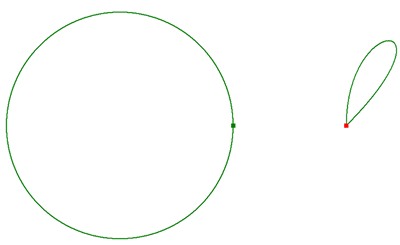﻿ How to build a polygon using segments and points—ArcObjects 10.4 Help for Java | ArcGIS for Desktop

# How to build a polygon using segments and points

### Building a polygon using segments and points

This example builds two multipart polygons in two different ways:
• The first polygon is built segment by segment.
• The second is built by defining its vertices as an array of WKSPoint structures.
The first way gives you the most control if you are using advanced construction techniques or curved segments (circular arcs, Bézier curves, and so on).
The second way is recommended for efficiently building polygons from bulk coordinate data that have vertices connected only with straight lines. The techniques shown here can also be applied to polyline construction. The first polygon should look something like the following illustration:See the following code example:
[Java]``` static void constructPolygons()throws Exception{ //Build a polygon segment by segment. //Create the segments and rings. If this is a single-part polygon you can add //segments directly to the polygon and it creates the ring internally. //You cannot reuse the same ring object. Also, when rings are added to the polygon //it takes ownership of them. You cannot reuse a ring for building another polygon. //These same restrictions also apply to segments. ICircularArc circularArc = new CircularArc(); IBezierCurveGEN bezierCurve = new BezierCurve(); ISegmentCollection ring1 = new Ring(); ISegmentCollection ring2 = new Ring(); ring1.addSegment((ISegment)circularArc, null, null); ring2.addSegment((ISegment)bezierCurve, null, null); IPolygon polygon = new Polygon(); //Always define the spatial reference of new top-level geometries. //polygon.SpatialReference = ... code skipped. IGeometryCollection geometryCollection = (IGeometryCollection)polygon; geometryCollection.addGeometry((IGeometry)ring1, null, null); geometryCollection.addGeometry((IGeometry)ring2, null, null); //At this point, you have constructed a _shell_ geometry. It consists of one //polygon containing two rings, each of which contains one segment. //However, the coordinates of those segments have not been defined. //Because you still have references to those segments, you can define their //coordinates now. //Circular arc. IPoint centerPoint = new Point(); centerPoint.setX( - 10); centerPoint.setY(0); circularArc.putCoordsByAngle(centerPoint, 0, 2 * Math.PI, 10); //Bézier curve. IPoint[] controlPoints = new IPoint; for (int i = 0; i < controlPoints.length; i++){ controlPoints[i] = new Point(); } controlPoints.setX(10); controlPoints.setY(0); controlPoints.setX(10); controlPoints.setY(10); controlPoints.setX(20); controlPoints.setY(10); controlPoints.setX(10); controlPoints.setY(0); bezierCurve.putCoords(controlPoints); //The polygon has now been defined. When changing segment coordinates directly //like this, you need to be careful to let the top-level geometry know that //things have changed underneath it so that it can delete any cached properties //that it might be maintaining, such as envelope, length, area, etc. //When you use certain methods on the top-level geometry implementation //of IGeometryCollection interface, like AddGeometry, it will automatically //invalidate any cached properties. geometryCollection.geometriesChanged(); //Build another polygon from a bunch of points. As before, assume that //two parts (rings) need to be created. If the polygon is a single part, you can //add the points directly to the polygon without creating a ring. IGeometryCollection pointPolygon = new Polygon(); //Define the spatial reference of the new pointPolygon here, code skipped. //As previously stated, the rings used for the first polygon cannot be reused. ring1 = new Ring(); ring2 = new Ring(); //At 9.2, the recommended way to add arrays of points to a geometry is to use //the IGeometryBridge2 interface on the GeometryEnvironment singleton object. IGeometryBridge2 geometryBridge = new GeometryEnvironment(); _WKSPoint[] wksPoints1 = new _WKSPoint[ring1.getSegmentCount()]; geometryBridge.setWKSPoints((IPointCollection4)ring1, wksPoints1); _WKSPoint[] wksPoints2 = new _WKSPoint[ring2.getSegmentCount()]; geometryBridge.setWKSPoints((IPointCollection4)ring2, wksPoints2); pointPolygon.addGeometry((IGeometry)ring1, null, null); pointPolygon.addGeometry((IGeometry)ring2, null, null); //The point polygon is now defined. } ```

Development licensingDeployment licensing
ArcGIS for Desktop BasicArcGIS for Desktop Basic
ArcGIS for Desktop StandardArcGIS for Desktop Standard